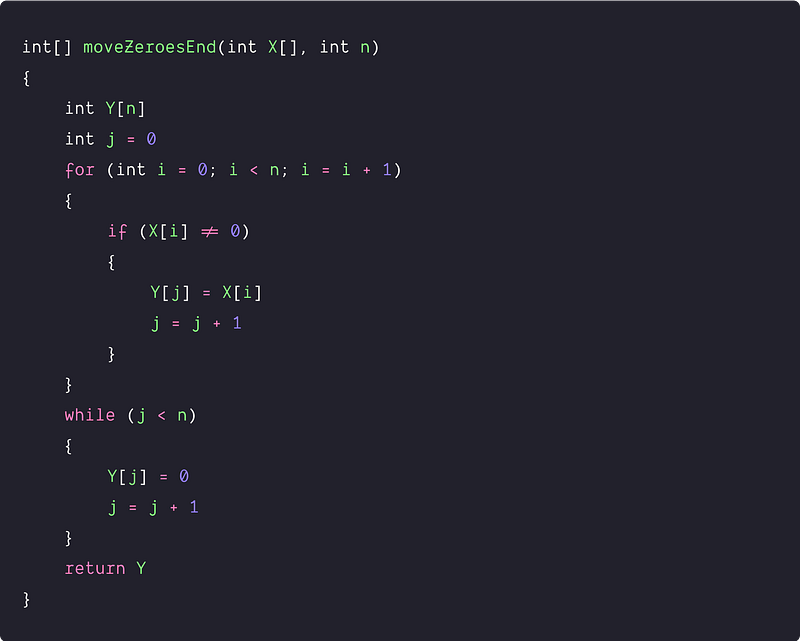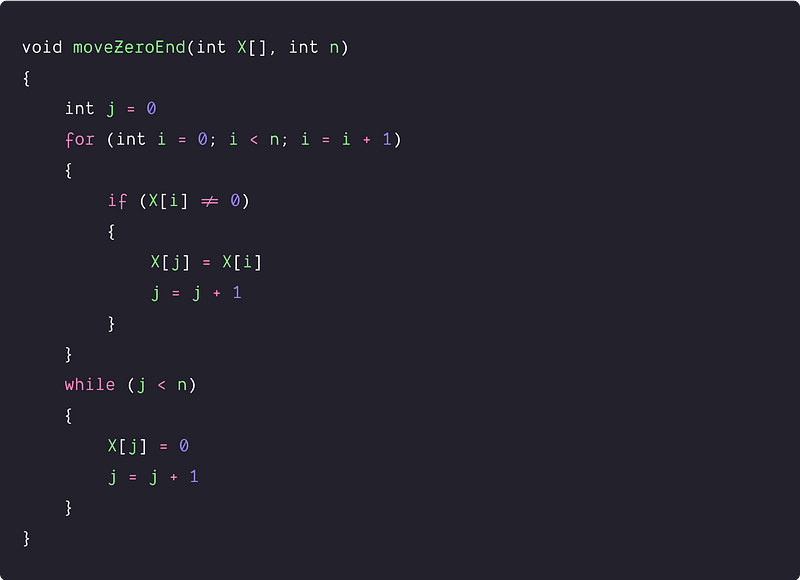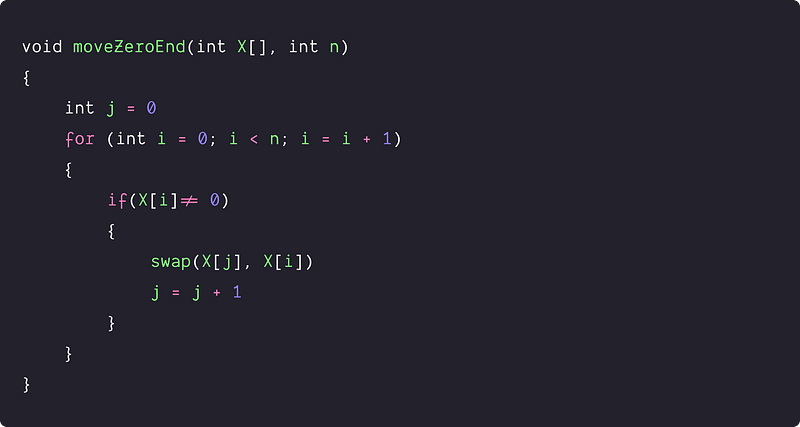# Move zeroes to end of an array

Key-takeaway: The efficient solution is a variation of two pointers approach where pointers are moving in the same direction. The solution idea is similar to the partition process of the quicksort.

### Let’s understand the problem

Given an array X[] of n elements filled with several integers, some of them being zeroes, write a program to move all the zeroes to the end.

Examples

Input: X[] = [4, 8, 6, 0, 2, 0, 1, 15, 12, 0], Output: [4, 8, 6, 2, 1, 15, 12, 0, 0, 0]

Input: X[] = [0, 3, 5, 9, 0, 0, 23, 2], Output: [3, 5, 9, 23, 2, 0, 0, 0]

Important Note: Before moving to the solutions, we recommend learners solve this problem. If solved, then well done! We would like to hear your ideas in the comment. Otherwise no problem, this is an opportunity to learn a new pattern in problem-solving!

Follow-up questions for the interviewer

• Candidate: Do we need to maintain the relative order of the elements in the output? Interviewer: Yes, we need to maintain the relative order of the non-zero elements.
• Candidate: Do we need to solve this problem in-place? Interviewer: Yes, we are looking for an O(n) in-place solution with the minimum number of operations.
• Candidate: Other than zero, are the input values unique? Interviewer: No, it can be repeated.

### Discussed solution approaches

1. A brute force approach  using extra space
2. Using two pointers: Double scan
3. An efficient approach using  two pointers: Single scan

### A brute force approach using extra space

Solution Idea

One basic idea would be to traverse the input array X[] and store all the non-zero elements in an extra memory Y[] of size n. Now fill the remaining empty space of Y[] with zeroes and return it as an output.

• Initialize two-pointers i and j where pointer i is for traversing the input array X[] and j is for storing values in Y[]. Both pointers will move in the same direction.
• During the traversal, when we find a non-zero element x[i], then store it in Y[j] and increment both i and j. Otherwise, increase the pointer i.

Solution PseudocodeTime and space complexity analysis

Time Complexity = Time complexity of traversing X[] and storing non-zero elements in Y[] + Time complexity of storing zeroes in Y[]  = O(n) + O(n) = O(n). In other words, we are doing a single traversal of both the array in the worst case.

Space Complexity = O(n), for the extra space Y[].

### Using two pointers: Double scan

Solution Idea and Steps

Now the critical question is :  can we further optimize the above algorithm to reduce the space complexity or solve the problem In-place? Let’s think!

We can use the above approach but rather than storing it in extra space, we can re-order the zero and non-zero elements in the input array itself. Here are the basic steps :

• Traverse the input array using two pointers i and j where i is for traversing the array and j is for tracking the last index of the non-zero element.
• Whenever we encounter a non-zero element then we shift it to the start of X[] i.e. if (X[i] != 0) then we update X[j] = X[i]. We also increment both i and j by 1. Otherwise, skip the zero elements and move the pointer i by 1.
• After the whole process, all elements in X[0…j-1] are non-zero elements. Now again traverse X[] from j to n-1 and fill it with zeroes. (Think!)

Solution PseudocodeTime and space complexity analysis

Time complexity (In the worst case) = Time complexity of traversing X[] to shift non-zero elements + Time complexity of traversing X[] to fill zeroes  = O(n) + O(n) = O(n). We are solving the problem In-place, so space complexity = O(1)

### An efficient approach using two pointers: Single scan

Solution idea

Can we further optimize the above approach and solve it in just one traversal? Can we reduce the second traversal to fill the zero in the end? Let's think!

In the above method, instead of assigning X[j] to X[i], if we swap both elements then we wouldn’t need to fill the rest of the array with zeroes in the end. All non-zero elements will be moved to the end due to swapping. (Think!)

Solution PseudocodeTime and space complexity analysis

We are doing only a single traversal where comparison and swapping are the critical operations. Time Complexity = O(n), Space Complexity = O(1)

Important Note: We recommend learners transform the above pseudo-codes into a favorite programming language (C, C++, Java, Python, etc.) and verify all the test cases. Please let us know if you find any errors or bugs; we would be highly grateful. Enjoy programming!

### Critical ideas to think!

• Using a similar approach, can we sort an array with only two values repeated? How will we solve this when three values are repeated?
• In the efficient approach, what would be the count of swap operations in the worst-case scenario?
• Can we solve this problem using some other approach? Can we use two pointers moving in the opposite direction?
• Will the above approaches preserve the relative order of non-zero elements?
• Explain the best and worst-case scenarios of all three approaches.
• In the 2nd approach, why are we traversing again to store zeroes to the end of the input array?

### Comparisons of Time and Space Complexities

• Brute force approach : Time = O(n), Space = O(n)
• Two pointers(Double Scan): Time = O(n), Space = O(1)
• Two pointers(Single Scan): Time = O(n), Space = O(1)

### Suggested coding questions to practice

• Double the first element and move zero to the end
• Segregate Even and Odd numbers
• Segregate 0s and 1s in an array
• Move all values equal to K to the end of the Array
• The minimum swaps required to sort the array in ascending order
• Sort an array in the waveform
• Remove duplicates from sorted array
• Sort an array of 0s, 1s and 2s

Enjoy learning, Enjoy coding, Enjoy algorithms!

Get well-designed application and interview centirc content on ds-algorithms, machine learning, system design and oops. Content will be delivered weekly.